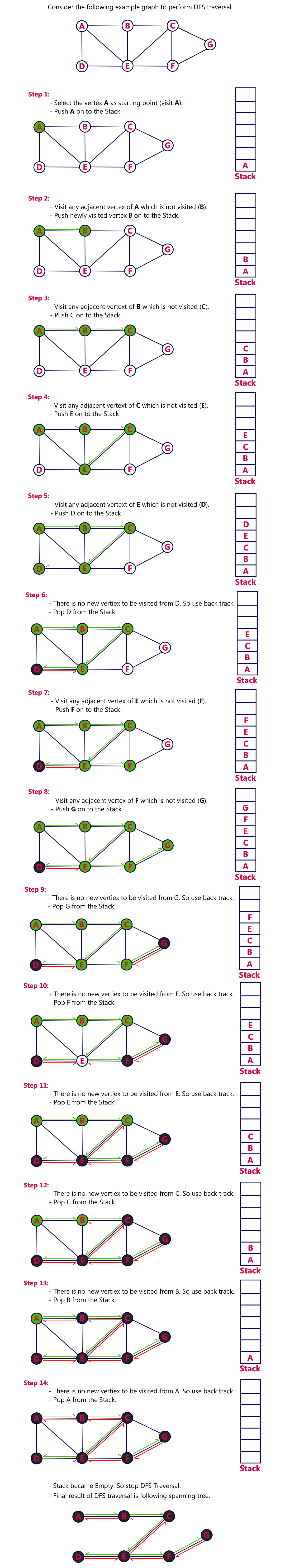The perfect place for easy learning...

×

# Graph Traversal - DFS

Graph traversal is a technique used for a searching vertex in a graph. The graph traversal is also used to decide the order of vertices is visited in the search process. A graph traversal finds the edges to be used in the search process without creating loops. That means using graph traversal we visit all the vertices of the graph without getting into looping path.

There are two graph traversal techniques and they are as follows...

1. DFS (Depth First Search)

# DFS (Depth First Search)

DFS traversal of a graph produces a spanning tree as final result. Spanning Tree is a graph without loops. We use Stack data structure with maximum size of total number of vertices in the graph to implement DFS traversal.

We use the following steps to implement DFS traversal...

• Step 1 - Define a Stack of size total number of vertices in the graph.
• Step 2 - Select any vertex as starting point for traversal. Visit that vertex and push it on to the Stack.
• Step 3 - Visit any one of the non-visited adjacent vertices of a vertex which is at the top of stack and push it on to the stack.
• Step 4 - Repeat step 3 until there is no new vertex to be visited from the vertex which is at the top of the stack.
• Step 5 - When there is no new vertex to visit then use back tracking and pop one vertex from the stack.
• Step 6 - Repeat steps 3, 4 and 5 until stack becomes Empty.
• Step 7 - When stack becomes Empty, then produce final spanning tree by removing unused edges from the graph

Back tracking is coming back to the vertex from which we reached the current vertex.

##### Example